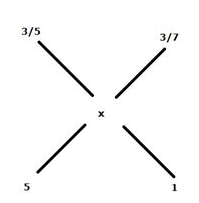# SBI Clerk Prelims 2021 Quantitative Aptitude Questions (Day-68)

Dear Aspirants, Our IBPS Guide team is providing new series of Quantitative Aptitude Questions for SBI Clerk Prelims 2020 so the aspirants can practice it on a daily basis. These questions are framed by our skilled experts after understanding your needs thoroughly. Aspirants can practice these new series questions daily to familiarize with the exact exam pattern and make your preparation effective.

Start Quiz

Simplification

Directions (01-05): What value should come in the place of (?) in the following questions?

1) 40 * 64 * ? = 582 – 12 * 67

A.13

B.18

C.8

D.1

E.21

2) 140% of 540 + 80% of ? = 4 * 829

A.2800

B.3000

C.3200

D.3400

E.3600

3) √1849 + ? = 8(2/3) * √2304

A.373

B.376

C.378

D.381

E.385

4) (124 ÷ 2728) * (90 ÷ 2430) * ? = 10

A.5940

B.4200

C.4800

D.5100

E.5300

5) (12167)(1/3) + (4913)(1/3) + √400 = ?

A.40

B.50

C.60

D.70

E.80

Profit and loss

6) Ratio of the cost price to marked price of the article is 4: 5. A shopkeeper offer a discount of 15% and the marked price of the article is Rs.720. What is the profit percentage of the article?

A.6.25%

B.4.75%

C.5.5%

D.3.40%

E.None of these

Mixture and allegation

7) Ratio of the mixture of milk and water in vessel A and B is 3: 2 and 3: 4 respectively. If both vessel A and B mixtures are mixed together in the ratio of 5: 1, then the quantity of the milk in the final mixture is approximately what percent more than the quantity of the water in the final mixture?

A.22%

B.33%

C.29%

D.44%

E.55%

Pipes and cistern

8) Pipe A can fill the tank in 40 hours and ratio of the efficiency of pipe A to B is 3:2. Pipe B and pipe C together can fill the tank in 30 hours. In how many hour pipe A, B and pipe C together can fill the tank?

A.13(1/7) hours

B.14(1/7) hours

C.15(1/7) hours

D.17(1/7) hours

E.None of these

Ages

9) Ratio of the ages of A and B is 4: 5 and after 4 years the ratio of the ages of B and C is 3:4. If the total sum of the ages of A, B and C is 64 years, what is the present age of B?

A.18 years

B.20 years

C.24 years

D.28 years

E.None of these

Probability

10) A basket contains x black, 4 red and 5 green colour balls. One ball is taken randomly and the probability of getting a green colour ball is 1/3, and then finds the total number of balls in the basket

A.12

B.14

C.15

D.18

E.20

40 * 64 * ? = 582 – 12 * 67

2560 * ? = 3364 – 804

? = 1

140% of 540 + 80% of ? = 4 * 829

756 + 80 % of ? = 3316

80% of ? = 2560

? = 3200

√1849 + ? = 8(2/3) * √2304

43 + ? = 26/3 * 48

43 + ? = 416

? = 373

(124 ÷ 2728) * (90 ÷ 2430) * ? = 10

1/22*1/27*?=10

? = 5940

(12167)(1/3) + (4913)(1/3) + √400 = ?

23 + 17 + 20 = ?

60 = ?

CP = 4/5 * 720 = Rs.576

SP = 720 * 85/100 = Rs.612

Profit percentage = (612 – 576)/576 * 100

= 6.25%

Milk in vessel A = 3/5

Water in vessel A = 2/5

Milk in vessel B = 3/7

Water in vessel B = 4/7(x – 3/7) / (3/5 – x) = 5/1

(7x – 3) * 5/(3 – 5x) * 7 = 5/1

7x – 3 = 21 – 35x

42x = 24

x = 4/7

Water in final mixture = 3/7

Required percentage = (4 – 3)/3 * 100 = 33%

The work done by A in one hour,

A = 1/40

The tank will be filed by B in,

B = 3/2 * 40 = 60 hours

Pipe B and pipe C together can fill the tank in 30 hours,

B + C = 1/30

C = 1/30 – 1/60

C = 1/60

A + B + C = 1/40 + 1/60 + 1/60

= 3 + 2 + 2/120

= 7/120

Required time = 120/7 hours = 17(1/7) hours

A + B + C = 64

A/B = 4/5

A = 4/5 B

B + 4/C + 4 = ¾

3C + 12 = 4B + 16

3C – 4B = 4

3C = 4B + 4

A + B + C = 64

4/5 B + B + (4B + 4)/3 = 64

12B + 15B + 20B + 20 = 64 * 15

47B = 940

B = 20

Given,

Total no. of probability = 9+x

Required probability = 5/(9+x)=1/3

=>9+x=15=>x=6

 Check Here to View SBI Clerk Prelims 2021 Quantitative Aptitude Questions Day – 67 Day – 66 Day – 65
4 1 vote
Rating